Frontiers journals are at the top of citation and impact metrics

# Frontiers in Applied Mathematicsand Statistics

## Biological Modeling and Simulation## Original Research ARTICLE

Front. Appl. Math. Stat., 31 May 2016 | https://doi.org/10.3389/fams.2016.00006

# Extended Topological Persistence and Contact Arrangements in Folded Linear Molecules

• 1Department of Mathematics, Stanford University, Stanford, CA, USA
• 2Department of Ophthalmology, Harvard Medical School, Harvard University, Boston, MA, USA

Structure plays a pivotal role in determining the functional properties of self-interacting linear biomolecular chains, for example proteins and nucleic acids. In this paper, we propose a method for representing each such molecule combinatorially—as a one-dimensional simplicial complex—in a novel way that takes into account intra-chain contacts. The representation allows for efficient quantification of structural similarities and differences between molecules, and for studying molecular topology using extended persistence. This method performs a multi-scale analysis on a filtered simplicial complex as it tracks clusters, holes, and higher dimensional voids in the filtration. From extended persistence we extract information about the arrangement of intra-chain interactions, a topological property which demonstrably affects folding and unfolding dynamics of the linear chains.

## 1. Introduction

Proteins and nucleic acids are linear organic polymers which are present in and vital to every living cell [1, 2]. They perform a vast array of functions within living organisms, for example, proteins provide mechanical support of cells, catalyze specific chemical reactions as well as transport and store nutrients and metabolites. In determining the functional properties of proteins and nucleic acids, and more generally, self-interacting molecular chains, shape plays a pivotal role. Chemists have been aware of the connection between the shape of a molecule and its function for decades already. For example, Linus Pauling wrote in 1974: “I am convinced that it will be found in the future…that the shapes and sizes of molecules are of just as great significance in determining their physiological behavior as are their internal structure and ordinary chemical properties .”

Topology is the branch of mathematics which deals with shape. It can be used to study connectivity information, which includes the classification of connected components of a space, loops and higher dimensional surfaces within the space. It can further be used to approximate complicated objects with simple, combinatorial ones, for example simplicial complexes. In the past decade with the emergence of ‘big data’, topology started playing a more prominent role in data analysis [4, 5]. Using a method called persistent homology  and a generalization of it, called extended persistence , researchers have solved problems in sensor networks [8, 9], gained insights into texture images  and classified lesions [11, 12]. Topological ideas have also inspired methods for visualizing complex datasets .

Chemists and structural biologists have successfully applied topological principles to classify elementary features in the structure of molecules . Knot theory provided an appropriate framework for modeling the structure and function of the genomic DNA and for the action of particular enzymes in altering the topology of DNA in site-specific recombination. However, physical knots are extremely rare in RNA molecules . Polypeptide chains can form knots , but only a small fraction of proteins (< 1%) in the Protein Data Bank (PDB), including rRNA methyltransferases, carbonic anhydrides, and ubiquitin hydrolase, are identified to be knotted [22, 23]. Although some progress has been made , knot theory does not suffice to distinguish between most RNA and protein folds.

Circuit topology  addresses this problem and takes into account multiple intra-molecular contacts (interactions), in which one part of the chain binds to another part. The arrangements of these contacts (parallel, series, cross; see Figure 1) can be used for classification of biomolecules; molecular structures that are not resolvable with previous methods may be distinguished based on their circuit topology . Circuit topology complements other contact based methods (e.g., contact order based approach, Gaussian network models, Anisotropic network models) in predicting folding kinetics and their relation to molecular shape . Recent studies revealed the power of circuit topology framework in determining the (un)folding dynamics  as well as functions  of folded (bio)polymers.

FIGURE 1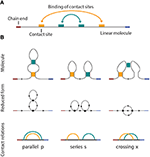Figure 1. (A) A linear molecule with four contact sites. The two chain ends are distinguishable in linear biomolecules like nucleic acids and proteins. The curved arrows show how the molecule folds, i.e., four contact sites merge into two binary contacts. The arrangement is parallel in this case, as shown in (B), on the left side. (B) Three basic circuit relations between two contacts in a linear molecule. From left to right: parallel, series, and crossed.

In this paper, we work within the circuit topology framework and provide a method for representing every linear molecule as a one-dimensional simplicial complex. While the idea of representing a molecule as a graph is not novel , our approach is, as it takes into account intra-molecular contacts in such a way that the circuit relations between contacts are preserved. As a measure of similarity and dissimilarity, we propose the graph edit distance which is commonly used in bioinformatics and structural biology for comparing structures . Once we have a metric space model for linear molecules, we can use a plethora of other tools, for example, compute persistent homology, extended persistence, etc. In this paper, in particular, we use extended persistence to extract complete information about contact arrangements. This is the first computational method available to compute arrangement matrices presented in Mashaghi et al. . Extended persistence has been successfully applied to analyze biomolecules previously , however this is the first time it has ever been used in the context of circuit topology.

## 2. Mathematical Background

The aim of this work is to analyze properties of self-interacting linear molecular chains using topological methods. We present the theoretical material in a compact, informal manner following [4, 5] and encourage the readers interested in details to read the given references. For a deep treatment of homology theory, we refer the reader to Allen Hatcher's Algebraic Topology .

### 2.1. Simplicial Complexes and Homology

The idea behind algebraic topology is that one can distinguish spaces by the occurrences of patterns within a space. Homology is one of many topological invariants that can be used for this purpose, but has the advantage of being relatively easy to compute. It allows us to assigns the so-called Betti numbers, i.e., a list of non-negative integers, β0, β1, β2, …, to any topological space. Each of these numbers carries connectivity information about the space, for example, β0 counts the number of connected components of a space, β1 counts the number of loops in the space, β2 counts the number of voids, etc.

Sometimes ignoring a certain amount of data or structure yields a simpler theory, which can give results not readily obtainable in the original setting. Relative homology is an example where this occurs. Given a space X and a nice enough subset A, the relative homology of (X, A), H(X, A), looks exactly like the homology of the quotient XA (except in dimension 0, where XA always has one connected component more). A quotient space XA is, intuitively speaking, the result of identifying points in X that belong to A.

Homology was initially defined for spaces described in a very particular way, namely as simplicial complexes. The basic idea of a simplicial complex is that of gluing together points, lines, triangles, tetrahedra, and the higher dimensional equivalents (which are called simplices) along their boundaries in a structured way.

We formally define a k-simplex as follows. Suppose that k + 1 points ${v}_{0},\dots ,{v}_{k}\in {ℝ}^{n}$ are affinely independent, i.e., v1v0, …, vkv0 are linearly independent vectors. Then the set of points

$C={t0v0+…+tkvk|ti≥0,0≤i≤k,∑i=0kti=1}.$

is a k-simplex. We denote it by {v0, …, vk}. A 0-simplex is a point or a vertex, a 1-simplex is an edge, a 2-simplex is a triangle and a 3-simplex is a tetrahedron (see Figure 2 for examples).

FIGURE 2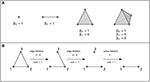Figure 2. (A) A vertex, an edge, a triangle, and a tetrahedron. In all three cases β0 = 1. There are no loops or higher dimensional voids and therefore βn = 0 for n ≥ 1. (B) Example of an edit path whose cost is 3. This is the minimal cost edit path, thus the graph edit distance between these two graphs is 3.

A simplicial complex K is a finite collection of simplices that satisfies the following conditions:

1. If σ ∈ K and a simplex τ ⊆ σ, then τ is also in K.

2. The intersection of any two simplices σ1, σ2K is either ∅ or a face of both σ1 and σ2.

1-dimensional simplicial complexes have been widely enough studied to deserve their own name, graphs, and the branch of mathematics that studies them is graph theory. A subcomplex of a simplicial complex K is an simplicial complex K′ ⊆ K.

### 2.2. Distance Functions on the Space of Graphs

Once we have a way to represent every linear molecule as a graph, it would be desirable to have a notion of “distance” on this space of molecules that would serve as a measure of how “similar” or “dissimilar” two molecules are. A space equipped with such a function (distance function) is called a metric space. Formally, a distance function d: X × X → ℝ on space X is a function that satisfies the following properties:

• For all x, yX, d(x, y) ≥ 0 and d(x, y) = 0 if and only if x = y.

• For all x, yX, d(x, y) = d(y, x) (symmetry).

• For all x, y, zX, d(x, y) ≤ d(x, z) + d(z, y) (triangle inequality).

One of the metrics commonly used on the space of all (finite) graphs is the graph edit distance [3943, 48] and its variations. Intuitively speaking, graph edit distance defines the similarity of two graphs by the minimum amount of distortion which is needed to transform one graph into the other.

Suppose we have a graph (V, Σ), where V denotes a finite set of vertices and Σ a finite set of edges between them. Each edge is represented by a two-element set that contains vertices that the edge connects. Let μ: V ∪ Σ → ℤ be a function that assigns an integer to each vertex and each edge. We call μ a labeling function. If the labeling function is not explicitly specified, we assume that all vertices have the same label. The allowed modifications include the insertion, deletion, and substitution of vertices and edges. A vertex deletion operation, for example, refers to the removal of a vertex from a graph, and a vertex/edge substitution operation is equivalent to changing the label of a vertex/edge substitution. A sequence of edit operations that transforms one graph into another is called an edit path. Computing the edit distance of two graphs is equivalent to finding an edit path with a minimal number of operations required.

The edit distance method can be tailored to a specific application by assigning each edit operation a cost that depends on the strength of the corresponding distortion. The total edit cost of a given edit path is the sum of costs of individual edit operations. The edit distance of two graphs is defined to be the minimal cost edit path between them, that is, the least expensive way to edit one graph into the other one. Such an edit distance is a metric if the cost functions is positive definite, symmetric and satisfies triangle inequality at the level of single edit operations . If the cost of all operations is c, then the graph edit distance is just the minimal number of modifications required times c. See Figure 2B for an example of the graph edit distance computation.

### 2.3. Extended Persistence

Homology provides information about the number of connected components, loops and higher dimensional voids that occur within a topological space. However, sometimes as a tool it is not powerful enough to make desired distinctions between structures. For example, Figure 4 depicts three simplicial complexes that arise from three qualitatively different arrangements of contacts, but whose Betti numbers are all the same. A variant of homology, called extended persistence , allows us to extract information about a simplicial complex K equipped with a function f: K → {1, 2, …, n} (filtering function). In our case, function f records the time when a simplex is added in a filtration. Depending on the nature of the problem, it can be any other function significant to K. For example, Aadcock et al.  constructed simplicial complexes from images of liver lesions and used grayscale values as the filter.

To determine the extended persistence of (K, f) with respect to the i-dimensional homology functor Hi with coefficients in a field, construct the following sequence of vector spaces and maps between them:

$0→Hi(K1)→Hi(K2)→⋯→Hi(Kn)=Hi(K) →Hi(K,Kn)→⋯→Hi(K,K1)=0$

Here Ki contains such simplices from K that f(σ) ⊂ {1, 2, …, i} for every σ ∈ Ki; Ki contains such simplices from K that f(σ) ⊂ {i, …, n} for every σ ∈ Ki. In our case H(X, A) is isomorphic to H(XA), except in the case of 0-dimensional homology (in that case β0(XA) = β0(X, A) + 1).

The idea behind this sequence is to grow the space from the bottom up; and then to relativize it from the top down and keep track of when i-dimensional voids appear and disappear in this sequence.

Figure 3 depicts a simplicial complex with labeled vertices. The first eight pictures show how simplices are added. The following eight pictures represent spaces isomorphic to relevant quotients. The 1-dimensional extended persistence keeps track of when each loop appears and disappears in this sequence.

FIGURE 3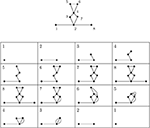Figure 3. A depiction of a simplicial complex with a filtering function (numbers next to vertices) and of spaces that appear in extended persistence. There are two loops of extended type—the first present over [6, 4) and the other over [7, 2). We interpret this as follows: in this simplicial complex there is one loop that begins to form at 2 and is completed at 7 and another completed at 6 whose first edge appears at 4.

The first stage or the standard part of the extended persistence is

$0→Hi(K1)→Hi(K2)→⋯Hi(Kn)$

while the second stage or the extended part is

$Hi(K,Kn)→Hi(K,Kn−1)→⋯→Hi(K,K1)=0.$

Every i-dimensional void which appears at some point of the two-stage process will eventually disappear. Depending on where in the extended filtration they appear and disappear, we distinguish three types of voids:

ordinary : appears at a and disappears at b during the first stage with a < b;

relative : appears at a and disappears at b during the second stage, with b < a;

extended : appears at a in one stage and disappears at b in the other.

In our setting we are only interested in the voids of the extended type. For example, in Figure 3, there are two loops of extended type—the first present over [6, 4) and the other over [7, 2). We interpret this as follows: in this simplicial complex there is one loop that begins to form at 2 and is completed at 7 and another completed at 6 whose first edge appears at 4. There are also several features of the relative type.

### 2.4. Circuit Topology

Pairwise relations between intramolecular contacts affect the function and properties of a molecule  (for example the folding rate of the molecule, etc). We distinguish three basic types of relations that can occur between two contacts: parallel, series, and cross. These three basic types and their simplicial complexes are depicted in Figure 4. Intuitively, the easiest way to determine the type of a relation is to consider the molecular strand as an interval of length one, and then check which points would bind together and form contacts. Figure 1 illustrates what happens for chains with two contacts. Each broken line connects two points that form a contact and from the picture it is easy to see what the “parallel” (denoted by p), “series” (denoted by s) and “cross” (denoted by x) arrangements refer to. This leads us to represent every contact as an interval [a, b] in which a is the point on a molecular chain that is linked to b (the molecular strand is directed and we take a < b).

FIGURE 4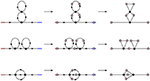Figure 4. Simplicial representations of molecules with parallel, series, and cross arrangement. In all three cases the corresponding Betti numbers are: β0 = 1, β1 = 2, βn = 0 for n ≥ 2.

We have a simple criterion to test these arrangements for interval representations. Let c1 = [a1, b1] and c2 = [a2, b2] be contacts. Without loss of generality we may assume that a1a2. Then:

c1 and c2 are parallel if [a2, b2] ⊆ [a1, b1];

c1 and c2 form a series if b1a2;

c1 and c2 cross if a2 < b1 < b2.

The arrangement of contact pair remains unchanged if we reverse the direction of the molecule. In terms of intervals this change would correspond to a map r: II, r(x) = 1 − x.

We record the information about the arrangements of these contacts in a n × n arrangement matrix, where n is the number of contacts and every element of the matrix is taken from {p, s, x}.

## 3. Results

### 3.1. Metric Space of Molecules

We first use the algebraic tools presented in the previous section to model the molecular chains with the most basic circuit topologies as simplicial complexes (Figure 4). In this paper we only consider chains with binary contacts as multi-valent contacts can typically be decomposed to multiple binary ones. In this setting each linear molecule can be thought of as directed chain with contacts linking different points on the chain. Red denotes the beginning of the chain and blue the end. Black dots are points of contact in the molecule and arrows denote the direction of the strand.

Algorithm 1 converts a linear molecule into a simplicial complex. We use it in Figure 5 to get a simplicial complex representation of the Hammerhead Ribozyme, a small RNA motif with catalytic activity capable of self-cleavage .

ALGORITHM 1
FIGURE 5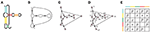Figure 5. (A) A depiction of Hammerhead Ribozyme and (B) a coarse grained schematic of the molecule. (C) The simplicial complex that represents Hammerhead Ribozyme. (D) Filter function of Hammerhead Ribozyme. Voids of extended type in the extended persistence are represented by a multiset {[15, 2), [7, 4), [16, 6), [14, 9), [13, 11)}. (E) Corresponding arrangement matrix. To arrive at the arrangement matrix, take the intervals representing voids of extended type and use the interval criterion from Section 2.4 to determine the pairwise arrangements of contacts.

We quantify the dissimilarity between two molecules as the graph edit distance between its simplicial complex (graph) representations. The simplest is to assign the same cost to all modifications. We can also tailor the cost value of a modification so that it reflects the strength of the corresponding distortion. In the case of linear molecules energetic and entropic factors come in. There are operations that are energetically forbidden (like breaking connectivity of the chain) to which we can assign greater cost. For intra-chain contacts, forming and breaking contacts are both possible, forming contacts being energetically favored over breaking. Depending on the context, we may take a graph edit distance that involves the least energy cost (and the highest energy release). Note that sequences of edge and vertex additions and deletions may result in graphs that are not representatives of linear molecules obtainable by using Algorithm 1, but this metric still provides a reasonable way of measuring the distance between the graphs that do.

In Figure 6, we calculate pairwise graph edit distances between three basic arrangements—parallel (P), cross (X) and series (S) arrangements. While other graphs may have identical topologies to the P-, S-, or X-type arrangements, it is these specific arrangments that represent linear molecules. The statistics of these arrangements defines folding kinetics; a molecule that is rich in S-type structures folds non-cooperatively while a molecule, which is rich in P-type structures, undergoes cooperative folding. Furthermore, inter-conversion of these structures represents a generic way through which a topology can evolve from another topology . As it turns out, the P- and S-arrangements are relatively close in distance, as are P- and X-arrangements, while X- and S-arrangements are relatively far.

FIGURE 6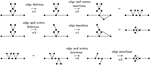Figure 6. The graph edit distance (with uniform costs) between a molecule with two contacts in series arrangement and a molecule with contacts in parallel arrangement is 4, as is the graph edit distance between a molecule with two contacts in parallel arrangement and a molecule with contacts in cross arrangement. The graph edit distance between molecules with contacts in series and cross arrangements is 8. It is not too difficult to see that the paths of distance 4 from P- to S-type arrangements and from S- to X-type arrangements are minimal, that the distance from an S- to an X-type arrangement is no less than 8 can be seen through detailed considerations of the possibilities of removing vertices (and edges) from a graph with 9 vertices and 10 edges (the S-type) to obtain a graph with 7 vertices and 8 edges (the X-type). For instance, removing two vertices connected to four edges each would already account for a graph edit distance of 10, so only certain vertices need to be considered.

### 3.2. On Genus

Bon et al.  have proposed the topological classification of RNA secondary structures with pseudoknots based on the concept of topological genus. The genus is a topological invariant, whose geometric interpretation is quite simple. For example, the genus of a graph is the minimal integer n such that the graph can be drawn without crossing itself on a sphere with n handles (i.e., an oriented surface of genus n). Computer software exists (for example SAGE) that can compute the genus of a given graph. So we can model an RNA molecules as graphs using Algorithm 1 (each graph is given as a list of vertices and 2-element sets of vertices determining the edges), find its genus and classify it according to Bon et al. , which demonstrates that our framework fits in well with other existing ones.

### 3.3. Determining the Contact Arrangement Matrix of a Molecule using Extended Persistence

Let M be any linear molecule. We can represent it as a simplicial complex K using Algorithm 1. As we move along the molecular strand we assign numbers to contacts—start with 1 for the beginning of the strand, 2 for the first point that binds to another point on the chain, etc. This defines a function f on K. See Figure 5D for such a filter function of the Hammerhead Ribozyme. The extended persistence of (K, f) is a multiset of intervals. We are interested in the voids of extended type {[bi, ai)}. Each interval carries information about a contact that connects two points on the molecular strand. For example, the appearance of [bi, ai) demonstrates that there is a contact completed at bi that starts forming at ai. Using the interval criterion from Subsection 2.4) we determine the pairwise arrangements of contacts, i.e., the arrangement matrix.

The software for computing extended persistence is available at http://www.mrzv.org/software/dionysus/.

### 3.4. Folding

Here we demonstrate the applicability of our proposed approach to study the folding process. Folding of a molecular chain involves sequential formation of contacts and starts with a configuration that includes no contact, the so called unfolded or extended state. The final product of this reaction is a natively folded state. Given a conformation with 2n-contact sites, which eventually forms n contacts with certain correct arrangement. At a certain time point we look at the system:

• it may have n′ contacts formed where n′ < n

• it may have n contacts formed.

We make use of the metric space model from Section 3.1 to represent folding schematically. The example we observe here is of pbu E adenine riboswitch [52, 53] and is depicted in Figure 7. Riboswitches are known to regulate genes through conformational changes in ligand-binding RNA aptamers [54, 55].

FIGURE 7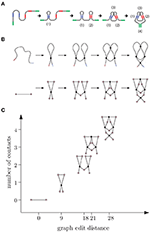Figure 7. (A) E adenine riboswitch folding. Four intramolecular contacts are formed during folding as indicated in the figure . (B) Coarse grained representation of the folding process. (C) We can represent folding in a graph where the x-axis (reaction coordinate) is the graph edit distance from the original molecule to the molecular strand with no contacts and y-axis is the number of contacts.

In the beginning, the molecule is a single chain with no intra-chain contacts. Then contacts start forming, increasing the complexity. Although the graph edit distance to a molecular chain with no contacts (represented by a graph with two vertices and an edge between them) increases with the number of contacts along this pathway, the relation between the number of contacts and changes in edit distance is not linear and and depends on how the topology changes during the transitions. We note that folding is not always a unidirectional path with increasing number of contacts. Sometimes the molecule adopts a non-native arrangement that has to be corrected before folding to native state is accomplished.

To further illustrate how topology changes during folding, we study some of the most basic folding and rearrangement steps. Figure 8 shows how graph edit distance to a molecular strand with no contacts changes upon formation of parallel contacts, the so-called zipping, series contacts and cross contacts. Graph edit distance to a molecular strand with no contacts is proportional to the number of contacts n and increases with a slope smaller, 7n, than that of series contacts, 9n. The approach presented here is general and can be applied to more complex molecular structures commonly seen in living organisms.

FIGURE 8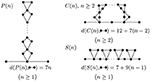Figure 8. Parallel, cross, and series arrangement in the case of n contacts. With each contact we add, the complexity increases and is detected by the graph edit distance.

## 4. Conclusions

Intra-chain interactions that bring two parts of a chain into physical contact are characteristic features of folded proteins and nucleic acids. The importance of these interactions has been widely recognized and numerous tools and approaches have been developed to identify the interactions and to relate them to molecular function and dynamics [36, 56]. Contact order and average connectivity of protein contact network have been successfully applied to explain folding rate and folding pathways of certain proteins [26, 36]. In the field of RNA research, a number of tools exist where graph theoretic approaches are used to characterize secondary and higher order structures. Graph edit distance is for example used by the Vienna RNA package [39, 40]. Despite all these efforts, there have been no tools available to extract arrangement of contacts from structures (beyond statistical measures e.g., contact order and average connectivity) and to efficiently compare the arrangements among many molecules or many conformations of one molecule.

In this paper we presented a systematic way of representing self-interacting linear molecules as simplicial complexes. We equipped the resulting spaces with a metric that can be used as a measure of similarity and dissimilarity between different molecules. One application of this model using extended persistence yields the first computational method for determining the contact arrangement matrix of a single chain molecule, which was previously determined manually . We can also use this model to structurally present RNA and protein folding processes, to analyze genome-wide chromosome conformation capture data, and to assess functional and evolutionary relatedness of biomolecules.

## Author Contributions

AM conceived and designed research. SK and AM performed the research and wrote the paper.

## Conflict of Interest Statement

The authors declare that the research was conducted in the absence of any commercial or financial relationships that could be construed as a potential conflict of interest.

The reviewer KX and handling Editor declared their shared affiliation and a past co-authorship and the handling Editor states that the process nevertheless met the standards of a fair and objective review.

## Acknowledgments

The authors thank Gunnar Carlsson for careful reading of the manuscript and helpful comments.

## References

1. Clancy S, Brown W. Translation: DNA to mRNA to Protein. Nat Educ. (2008) 1:101. Available online at: http://www.nature.com/scitable/topicpage/translation-dna-to-mrna-to-protein-393

2. Mashaghi A, Katan A. A physicist's view of DNA. De Physicus (2013) 3:59–61. Available online at: http://ceesdekkerlab.tudelft.nl/wp-content/uploads/Alireza-Allard-manuscript.pdf

3. Pauling L. The molecular basis of biological specificity. Nature (1974) 248:769–71.

4. Carlsson G. Topology and data. Bull Am Math Soc. (2009) 46:255–308. doi: 10.1090/S0273-0979-09-01249-X

5. Carlsson G. Topological pattern recognition for point cloud data. Acta Numerica (2013) 23:289–368. doi: 10.1017/S0962492914000051

6. Carlsson G, Zomorodian A. Computing persistent homology. Discrete Comput Geom. (2005) 33:249–74. doi: 10.1007/s00454-004-1146-y

7. Cohen-Steiner D, Edelsbrunner H, Harer J. Extending persistence using Poincaré and Lefschetz Duality. Found Comput Math. (2009) 9:79–103. doi: 10.1007/s10208-008-9027-z

8. Ghrist R, de Silva V. Coordinate-free coverage in sensor networks with controlled boundaries via homology. Int J Rob Res. (2006) 25:1205–22. doi: 10.1177/0278364906072252

9. Adams H, Carlsson G. Evasion paths in mobile sensor networks. Int J Rob Res. (2014) 34:90–104. doi: 10.1177/0278364914548051

10. Perea JA, Carlsson G. A Klein-bottle-based dictionary for texture representation. Int J Comput Vis. (2014) 1:75–97. doi: 10.1007/s11263-013-0676-2

11. Aadcock A, Rubin D, Carlsson G. Classification of hepatic lesions using the matching metric. Comput Vis Image Underst. (2014) 121:36–42. doi: 10.1016/j.cviu.2013.10.014

12. Ferri M, Stanganelli I. Size functions for the morphological analysis of melanocytic lesions. Int J Biomed Imaging (2010) 2010:621357. doi: 10.1155/2010/621357

13. Singh G, Mémoli F, Carlsson G. Topological methods for the analysis of high dimensional data sets and 3D object recognition. In Eurographics Symposium on Point Based Graphics, European Association for Computer Graphics. Prague (2007).

14. Brown D. Topology and chemistry. Struct Chem. (2002) 13:339–55. doi: 10.1023/A:1015872125545

15. Flapan E. When Topology Meets Chemistry: A Topological Look at Molecular Chirality. Cambridge; New York, NY; Washington, DC: Cambridge University Press (2000). doi: 10.1017/CBO9780511626272. Available online at: http://scholarship.claremont.edu/pomona_facbooks/30/

CrossRef Full Text

16. Tezuka Y, Oike H. Topological polymer chemistry. Prog Polym Sci. (2002) 7:1069–122. doi: 10.1016/S0079-6700(02)00009-6

17. Andersen JE, Penner RC, Reidys CM, Waterman MS. Topological classification and enumeration of RNA structures by genus. J Math Biol. (2013) 67:1261–78. doi: 10.1007/s00285-012-0594-x

18. Micheletti C, Di Stefano M, Orlandb H. Absence of knots in known RNA structures. Proc Natl Acad Sci USA. (2015) 112:2052–7. doi: 10.1073/pnas.1418445112

19. Mallam LA, Rogers JM, Jackson SE. Experimental detection of knotted conformations in denatured proteins. Proc Natl Acad Sci USA. (2010) 107:8189–94. doi: 10.1073/pnas.0912161107

20. Noel JK, Onuchic JN, Sulkowska JI. Knotting a protein in explicit solvent. Biophys J. (2014) 106:472a–3a. doi: 10.1016/j.bpj.2013.11.2672

21. Škrbić T, Micheletti C, Faccioli P. The role of non-native interactions in the folding of knotted proteins. PLoS Comput Biol. (2012) 8:e1002504. doi: 10.1371/journal.pcbi.1002504

22. Sulkowska JI, Rawdon EJ, Millett KC, Onuchic JN, Stasiak A. Conservation of complex knotting and slipknotting patterns in proteins. Proc Natl Acad Sci USA. (2012) 109:e1715–23. doi: 10.1073/pnas.1205918109

23. Virnau P, Mirny LA, Kardar M. Intricate knots in proteins: function and evolution. PLoS Comput Biol. (2006) 9:e122. doi: 10.1371/journal.pcbi.0020122

24. Rawdon EJ, Millett KC, Stasiak A. Subknots in ideal knots, random knots, and knotted proteins. Sci Rep. (2015) 5:90–104. doi: 10.1038/srep08928

25. Mashaghi A, van Wijk RJ, Tans SJ. Circuit topology of proteins and nucleic acids. Structure (2014) 22:1227–37. doi: 10.1016/j.str.2014.06.015

26. Baker D. A surprising simplicity to protein folding. Nature (2000) 405:39–42. doi: 10.1038/35011000

27. Pfleger C, Gohlke H. Efficient and robust analysis of biomacromolecular flexibility using ensembles of network topologies based on fuzzy noncovalent constraints. Structure (2013) 21:1725–34. doi: 10.1016/j.str.2013.07.012

28. Xia K, Wei GW. Persistent homology analysis of protein structure, flexibility, and folding. Int J Num Methods Biomed Eng. (2014) 30:814–44. doi: 10.1002/cnm.2655

29. Atilgan AR, Durell SR, Jernigan RL, Demirel MC, Keskin O, Bahar I. Anisotropy of fluctuation dynamics of proteins with an elastic network model. Biophys J. (2001) 80:505–15. doi: 10.1016/S0006-3495(01)76033-X

30. Yang L, Song G, Jernigan RL. Protein elastic network models and the ranges of cooperativity. Proc Natl Acad Sci USA. (2009) 106:12347–52. doi: 10.1073/pnas.0902159106

31. Kelin X, Zhixiong Z, Guo-Wei W. Multiresolution topological simplification. J Comput Biol. (2015) 22:887–91. doi: 10.1089/cmb.2015.0104

32. Mugler A, Tans SJ, Mashaghi A. Circuit topology of self-interacting chains: implications for folding and unfolding dynamics. Phys Chem Chem Phys. (2014) 16:22537–44. doi: 10.1039/C4CP03402C

33. Mashaghi A, Ramezanpour A. Distance measures and evolution of polymer chains in their topological space. Soft Matter (2015) 11:6576–85. doi: 10.1039/C5SM01482D

34. Mashaghi A, Ramezanpour A. Circuit topology of linear polymers: a statistical mechanical treatment. RSC Adv. (2015) 5:51682–9. doi: 10.1039/C5RA08106H

35. Nikoofard N, Mashaghi A. Topology sorting and characterization of folded polymers using nano-pores. Nanoscale (2016) 8:4643–9. doi: 10.1039/C5NR08828C

36. Dokholyan NV, Li L, Ding F, Shakhnovich EI. Topological determinants of protein folding. Proc Natl Acad Sci USA. (2002) 9:8637–41. doi: 10.1073/pnas.122076099

37. Ding CHQ, Meraz RF, He X, Holbrook SR. Contraction graphs for representation and analysis of RNA secondary structure. In: Proceedings of the 2004 IEEE Computational Systems Bioinformatics Conference. Stanford, CA (2004). pp. 716–7.

Google Scholar

38. Kim N, Fuhr K, Schlick T. Graph applications to RNA structure and function. In: Russell R, editor. Biophysics of RNA Folding. Vol. 3 of Biophysics for the Life Sciences. New York, NY: Springer (2013). pp. 23–51.

PubMed Abstract

39. Hofacker IL. RNA secondary structure analysis using the Vienna RNA package. Curr Protoc Bioinform. (2009) 26:12.2.1–16. doi: 10.1002/0471250953.bi1202s26

40. Lorenz R, Bernhart SH, zu Siederdissen CH, Tafer H, Flamm C, Stadler PF, et al. Vienna RNA Package 2.0. Algorithms Mol Biol. (2011) 6:26. doi: 10.1186/1748-7188-6-26

CrossRef Full Text

41. Akutsu T. Tree edit distance problems: algorithms and applications to bioinformatics. IEICE Trans Inform Syst. (2010) E93-D:208–18. doi: 10.1587/transinf.E93.D.208

42. Backofen R, Chen S, Hermelin D, Landau GM, Roytberg MA, Weimann O, et al. Locality and gaps in RNA comparison. J Comput Biol. (2007) 14:1074–87. doi: 10.1089/cmb.2007.0062

43. Khaladkar M, Bellofatto V, Wang JTL, Tian B, Shapiro BA. RADAR: a web server for RNA data analysis and research. Nucleic Acids Res. (2007) 35:1074–87. doi: 10.1093/nar/gkm253

44. Xia K, Wei GW. Persistent homology analysis of protein structure, flexibility, and folding. Int J Num Methods Biomed Eng. (2014) 30:814–44. doi: 10.1002/cnm.2655

45. Gameiro M, Hiraoka Y, Izumi S, Kramar M, Mischaikow K, Nanda V. A topological measurement of protein compressibility. Jpn J Ind Appl Math. (2015) 32:1–17. doi: 10.1007/s13160-014-0153-5

46. Sridharamurthy R, Doraiswamy H, Patel S, Varadarajan R, Natarajan V. Extraction of robust voids and pockets in proteins. In: EuroVis - Short Papers. Leipzig: The Eurographics Association (2013).

47. Hatcher A. Algebraic Topology. Cambridge, UK: Cambridge University Press (2002).

Google Scholar

48. Gao X, Xiao B, Tao D, Li X. A survey of graph edit distance. Pattern Anal Appl. (2010) 13:113–29. doi: 10.1007/s10044-008-0141-y

49. Bunke H, Allermann G. Inexact graph matching for structural pattern recognition. Pattern Recognit Lett. (1983) 1:245–53. doi: 10.1016/0167-8655(83)90033-8

50. Birikh KR, Heaton PA, Eckstein F. The structure, function and application of the hammerhead ribozyme. Eur J Biochem. (1997) 245:1–16.

51. Bon M, Vernizzi G, Orland H, Zee A. Topological classification of RNA structures. J Mol Biol. (2008) 379:900–11. doi: 10.1016/j.jmb.2008.04.033

52. VanLoock MS, Harris JM, Harvey SC. To knot or not to knot? Examination of 16S ribosomal RNA models. J Biomol Struct Dyn. (1998) 16:709–13. doi: 10.1080/07391102.1998.10508282

53. Silverman SK. A forced march across an RNA folding landscape. Chem Biol. (2008) 15:211–13. doi: 10.1016/j.chembiol.2008.02.014

54. Greenleaf WJ, Frieda KL, Foster DAN, Woodside MT, Block SM. Direct observation of hierarchical folding in single riboswitch aptamers. Science (2008) 319:630–3. doi: 10.1126/science.1151298

55. Nozinovic S, Reining A, Kim Y-B, Noeske J, Schlepckow K, Wöhnert J, et al. The importance of helix P1 stability for structural pre-organization and ligand binding affinity of the adenine riboswitch aptamer domain. RNA Biol. (2014) 11:655–66. doi: 10.4161/rna.29439

56. Chechetkin VR, Lobzin VV. Study of correlations in segmented DNA sequences: application to structure coupling between Exons and Introns. J Theor Biol. (1998) 190:69–83. doi: 10.1006/jtbi.1997.0535

Keywords: persistent homology, extended persistence, computational topology, biomolecular structure, circuit topology, folding

Citation: Verovšek SK and Mashaghi A (2016) Extended Topological Persistence and Contact Arrangements in Folded Linear Molecules. Front. Appl. Math. Stat. 2:6. doi: 10.3389/fams.2016.00006

Received: 30 December 2015; Accepted: 13 May 2016;
Published: 31 May 2016.

Edited by:

Guowei Wei, Michigan State University, USA

Reviewed by:

Matt Rathbun, California State University, Fullerton, USA
Kelin Xia, Michigan State University, USA

Copyright © 2016 Verovšek and Mashaghi. This is an open-access article distributed under the terms of the Creative Commons Attribution License (CC BY). The use, distribution or reproduction in other forums is permitted, provided the original author(s) or licensor are credited and that the original publication in this journal is cited, in accordance with accepted academic practice. No use, distribution or reproduction is permitted which does not comply with these terms.

*Correspondence: Alireza Mashaghi, alireza_mashaghi-tabari@meei.harvard.edu